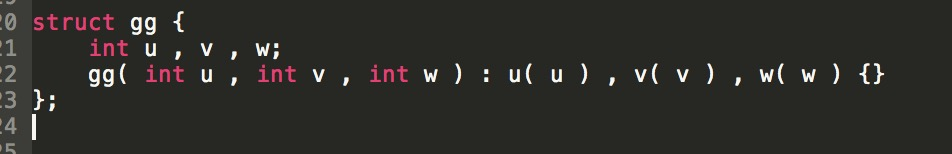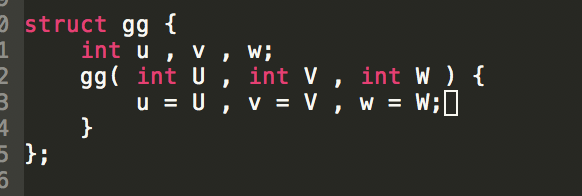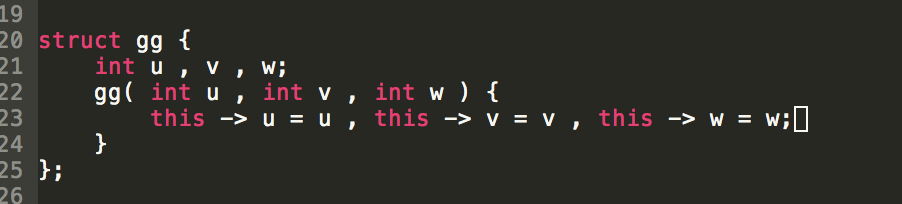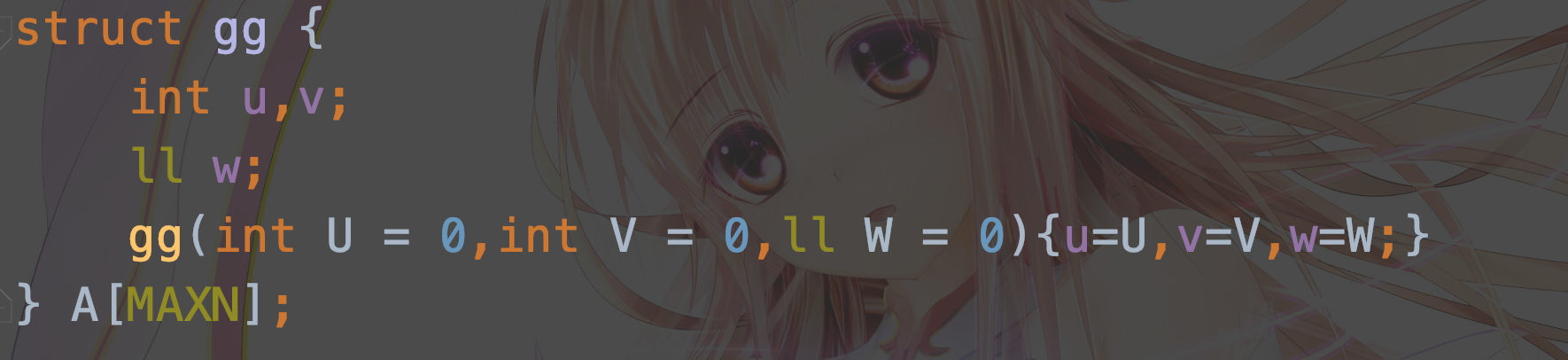# 语法上的小trick

## 构造函数## 快速读入

inline char nc(){
static char buf,*p1=buf,*p2=buf;
}
char ch=nc();int sum=0;
while(!(ch>='0'&&ch<='9'))ch=nc();
while(ch>='0'&&ch<='9')sum=sum*10+ch-48,ch=nc();
return sum;
}


_read()函数返回的就是读入信息。

## 迭代器

int a[] = {1,2,3};
set<int> s;
set<int>::iterator it;
s.insert(a,a+3);
for(it=s.begin();it!= s.end() ; ++it)cout<<*it<<" ";


## 重载运算符

inline matrix operator + (matrix &a, matrix &b) {//传参直接传指针，不需要拷贝，跑的更快。
static matrix res;//static创建了一个只能在此函数内使用的全局变量，使每次调用函数不用都开空间，节省时间。
rep(i) rep(j) res.array[i][j] = inc(a.array[i][j], b.array[i][j]);
return res;
}posted @ 2019-08-08 08:33  GavinZheng  阅读(138)  评论(0编辑  收藏